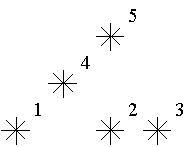Poj（2352）——Stars（树状数组）

Description

Astronomers often examine star maps where stars are represented by points on a plane and each star has Cartesian coordinates. Let the level of a star be an amount of the stars that are not higher and not to the right of the given star. Astronomers want to know the distribution of the levels of the stars.For example, look at the map shown on the figure above. Level of the star number 5 is equal to 3 (it's formed by three stars with a numbers 1, 2 and 4). And the levels of the stars numbered by 2 and 4 are 1. At this map there are only one star of the level 0, two stars of the level 1, one star of the level 2, and one star of the level 3.

You are to write a program that will count the amounts of the stars of each level on a given map.

Input

The first line of the input file contains a number of stars N (1<=N<=15000). The following N lines describe coordinates of stars (two integers X and Y per line separated by a space, 0<=X,Y<=32000). There can be only one star at one point of the plane. Stars are listed in ascending order of Y coordinate. Stars with equal Y coordinates are listed in ascending order of X coordinate.

Output

The output should contain N lines, one number per line. The first line contains amount of stars of the level 0, the second does amount of stars of the level 1 and so on, the last line contains amount of stars of the level N-1.

Sample Input

`51 15 17 13 35 5`

Sample Output

`12110`

``#include<stdio.h>#include<string.h>#include<iostream>#include<algorithm>using namespace std;#define maxn 32222//这道题目很巧妙，首先是根据x的增长来的，然后再根据y的增长来排的； //所以这样就能理解为什么每次就只要求x之前的就好了 int c[maxn];int levels[maxn];int lowbit(int x){	return x&(-x);}//这里是对前x进行求和； int sum(int x){	int res=0;	while(x>0){	//注意这里是x>0,不能写成x>=0; 		res+=c[x];		x-=lowbit(x);	}	return res;}//这里的目的是给下标为pos的加上1,这样的话下次询问前面的就能够累加上去了； void update(int pos){	while(pos<=32001){		c[pos]++;		pos+=lowbit(pos);	}}int main(){	int n,x,y;	while(~scanf("%d",&n)){		memset(levels,0,sizeof(levels));		int tt=n;		while(tt--){			scanf("%d%d",&x,&y);			levels[sum(x+1)]++;			update(x+1);		}		for(int i=0;i<n;i++) printf("%d\n",levels[i]);	}}``

## 关于作者白红宇是个全栈工程师，前端vue，小程序，app开发到后端框架设计，数据库设计，环境部署上线运维。

## 最新文章

Ajax的使用 2021-09-27
xshell 5 过期解决方案 2021-09-27

collatz()的函数 2021-09-27